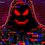# Aleph ($\aleph$) and Omega ($\omega$) Numbers Definition

Aleph-Null ($\aleph_0$) is the cardinality of the set of the natural numbers and Omega ($\omega$) is the infinite ordinal corresponding to that cardinal. But $\aleph_0+1$ still equals $\aleph_0$ while $\omega+1$ does not equal $\omega$! I get this when I read about Hilbert's Hotel. Same with that $2\aleph_0=\aleph_0$ so the cardinality of the set of integers is the same as the set of natural numbers. It is also the same as the set of rational numbers (see An easy proof that rational numbers are countable)! However the cardinality of the set of irrational and real and imaginary (probably, because a pure imaginary number is just $xi$, where $x$ is a real number and $i$ is the imaginary unit) and complex numbers is $\aleph_1$ and the ordinal that corresponds to it is $\omega_1$! The Aleph and Omega numbers do continue on.

My Questions:

1. What is the definition of $\aleph_2$ or $\aleph_3$ or $\aleph_4$ or just any $\aleph_n$ for that matter other than 'It's the next biggest infinite cardinal'?
2. What is $\omega_1-\omega$? $\omega_2-\omega_1$? Or just any $\omega_n-\omega_{n-1}$?Note by Lâm Lê
8 months, 1 week ago

This discussion board is a place to discuss our Daily Challenges and the math and science related to those challenges. Explanations are more than just a solution — they should explain the steps and thinking strategies that you used to obtain the solution. Comments should further the discussion of math and science.

When posting on Brilliant:

• Use the emojis to react to an explanation, whether you're congratulating a job well done , or just really confused .
• Ask specific questions about the challenge or the steps in somebody's explanation. Well-posed questions can add a lot to the discussion, but posting "I don't understand!" doesn't help anyone.
• Try to contribute something new to the discussion, whether it is an extension, generalization or other idea related to the challenge.
• Stay on topic — we're all here to learn more about math and science, not to hear about your favorite get-rich-quick scheme or current world events.

MarkdownAppears as
*italics* or _italics_ italics
**bold** or __bold__ bold
- bulleted- list
• bulleted
• list
1. numbered2. list
1. numbered
2. list
Note: you must add a full line of space before and after lists for them to show up correctly
paragraph 1paragraph 2

paragraph 1

paragraph 2

[example link](https://brilliant.org)example link
> This is a quote
This is a quote
    # I indented these lines
# 4 spaces, and now they show
# up as a code block.

print "hello world"
# I indented these lines
# 4 spaces, and now they show
# up as a code block.

print "hello world"
MathAppears as
Remember to wrap math in $$ ... $$ or $ ... $ to ensure proper formatting.
2 \times 3 $2 \times 3$
2^{34} $2^{34}$
a_{i-1} $a_{i-1}$
\frac{2}{3} $\frac{2}{3}$
\sqrt{2} $\sqrt{2}$
\sum_{i=1}^3 $\sum_{i=1}^3$
\sin \theta $\sin \theta$
\boxed{123} $\boxed{123}$

## Comments

Sort by:

Top Newest

I read that there are three of these ‘alephs’,and $\aleph _2$ stands for the number of all geometric curves.

- 8 months, 1 week ago

Log in to reply

Also note that the number of complex numbers is equal to the number of dots on a plane.

- 8 months, 1 week ago

Log in to reply

I remember a video I watched a while ago that said the ω's continue, each one infinitely bigger than the last. Same goes for the ℵ's. That means one minus the last is what you started with.

- 7 months ago

Log in to reply

×

Problem Loading...

Note Loading...

Set Loading...